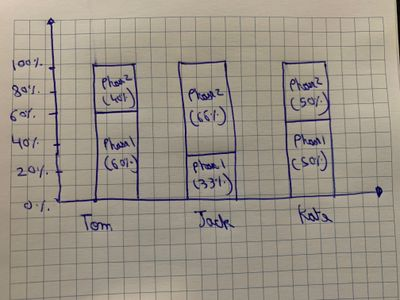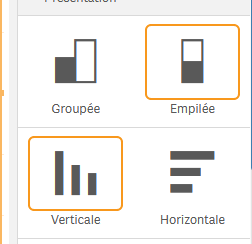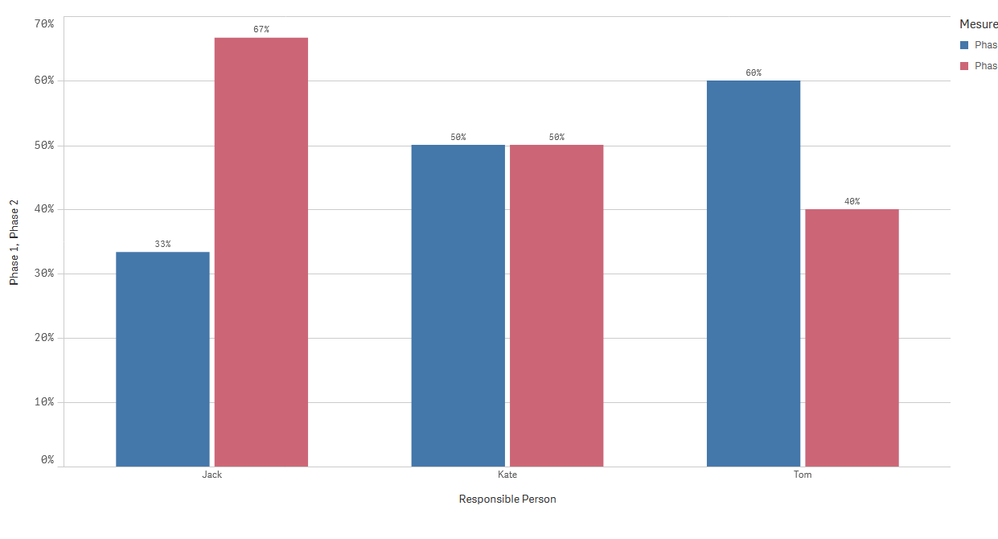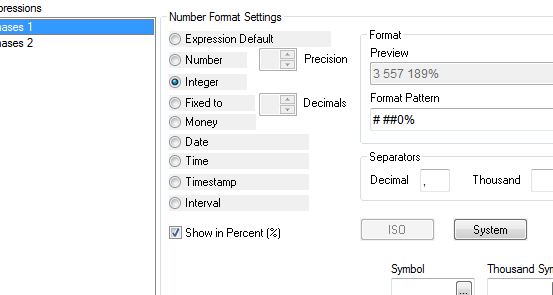# New to Qlik Sense

Discussion board where members can get started with Qlik Sense.

Announcements
BI & Data Trends 2021. Discover the top 10 trends emerging in today. Join us on Dec. 8th REGISTER
cancel
Showing results for
Did you mean:
HighlightedContributor III

## Percentage on Y axis for a bar chart using Set Analysis and Total function

Responsible Person, Phases, Value
Tom, Phase 1, 30
Tom, Phase 2, 20
Tom, Phase 3, 50
Jack, Phase 1, 10
Jack, Phase 2, 20
Jack, Phase 3, 10
Jack, Phase 4, 25
Kate, Phase 1, 10
Kate, Phase 2, 10
Kate, Phase 3, 50

]

Hi guys,
Here's a sample data that I have.
I just want to consider sum(Phase 1 and Phase 2) and present the numbers in percentage (Y- Axis)

I want to create a bar chart like this.How can I do it?

I believe we have to use Total function and Set Analysis.

Also, in the real data set, I have around 100 phases and I just have to display 5 out of it.

Thank you so much,

N

Labels (5)

• ### Total

1 Solution

Accepted Solutions
HighlightedMVP

@SkitzN27  if you want it in qlikview do below. No need to create additional measure. single measure is enough

```Dimensions:
1) Responsible Person
2) Phases

Expression:
=sum({<Phases={'Phase 1','Phase 2'}>}Value)/sum(TOTAL <[Responsible Person]>{<Phases={'Phase 1','Phase 2'}>}Value)```

Go to chart properties->Style-> select "stacked" option

14 Replies
HighlightedCreator II

Bar Chart; Dimension : [Responsible Person]

Measures :

Measure 1 = sum({< Phases={'Phase 1'}>}  Value) / sum({< Phases={'Phase 1','Phase 2','Phase 3','Phase 4','Phase 5'}>}  Value)

Measure 2 = sum({< Phases={'Phase 2'}>}  Value) / sum({< Phases={'Phase 1','Phase 2','Phase 3','Phase 4','Phase 5'}>}  Value)

Measure 3 = sum({< Phases={'Phase 3'}>}  Value) / sum({< Phases={'Phase 1','Phase 2','Phase 3','Phase 4','Phase 5'}>}  Value)

etc...

measure display in %

HighlightedMaster

@SkitzN27  for example in dimension Responsible Person

and measure 1:Phase1 :

=sum({<Phases={'Phase 1'}>}Value)/(sum({<Phases={'Phase 2'}>}Value)+sum({<Phases={'Phase 1'}>}Value))

measure 2: phase 2

=sum({<Phases={'Phase 2'}>}Value)/(sum({<Phases={'Phase 2'}>}Value)+sum({<Phases={'Phase 1'}>}Value))

change formating number to Number->pourcentage

and change bar chart style to :outpout:orRegards,
Taoufiq ZARRA

"Please LIKE posts and "Accept as Solution" if the provided solution is helpful "

(you can mark up to 3 "solutions") 😉
HighlightedMVP

@SkitzN27  Create a bar chart like below

``````Dimensions:
1) Responsible Person
2) Phases

Expression:
=sum({<Phases={'Phase 1','Phase 2'}>}Value)/sum(TOTAL <[Responsible Person]>{<Phases={'Phase 1','Phase 2'}>}Value)``````

Go to Appearance->Presentation and select  "Stacked" option

HighlightedContributor III

Hi Taoufiq,

Thanks so much.

I have to do it in Qlikview, how can I change it to percentage there?

Also, is there any other way to write the denominator rather than having to write sum statement two times in denominator. ( (sum({<Phases={'Phase 2'}>}Value)+sum({<Phases={'Phase 1'}>}Value)))

HighlightedMaster

@SkitzN27  attached Qlikview file

=sum({<Phases={'Phase 1'}>}Value)/(sum({<Phases={'Phase 2','Phase 1'}>}Value))

for pourc.Regards,
Taoufiq ZARRA

"Please LIKE posts and "Accept as Solution" if the provided solution is helpful "

(you can mark up to 3 "solutions") 😉
HighlightedMVP

@SkitzN27  if you want it in qlikview do below. No need to create additional measure. single measure is enough

```Dimensions:
1) Responsible Person
2) Phases

Expression:
=sum({<Phases={'Phase 1','Phase 2'}>}Value)/sum(TOTAL <[Responsible Person]>{<Phases={'Phase 1','Phase 2'}>}Value)```

Go to chart properties->Style-> select "stacked" option

HighlightedContributor III

Thanks Kush,

this looks great and is very optimized but in this case, how do I present the numbers and percentage on the bar chart like this in Qlikview?

It seems like Qlikview doesn't have that option.HighlightedContributor III

Thanks so much, it does work.
Although, I'd like to display numbers on the bar ( not the percentage).

You think that's possible?

Something like this maybe-N

HighlightedMVP

@SkitzN27  see the attached